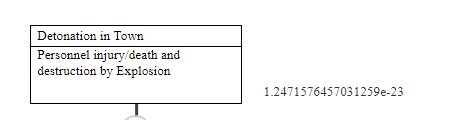# Convert value to scientific notation for negative powers

Mylau shared this problem 2 years ago
In Progress

I was provided a prototype that can convert numerical values into scientific notation for positive powers (ex. 3000 -> 3.00 x 10^3). However, my prototype involves only small values (such as 0.0003) and needs to work for negative powers of 10, I tried modifying the given prototype but failed.

The current prototype works by extracting the value (first three values) from input using substring function, add decimal and 'E' using concat, and add power using count function (input-1).

Since in case of small decimals (ex. 0.0003), the first number to retain will no longer be in the front, I tried to make use of the index function to root out the placement of the first non-zero (also not decimal) value, but as you can see on the pic below, it didn't work saying the highlighted expression is comparative.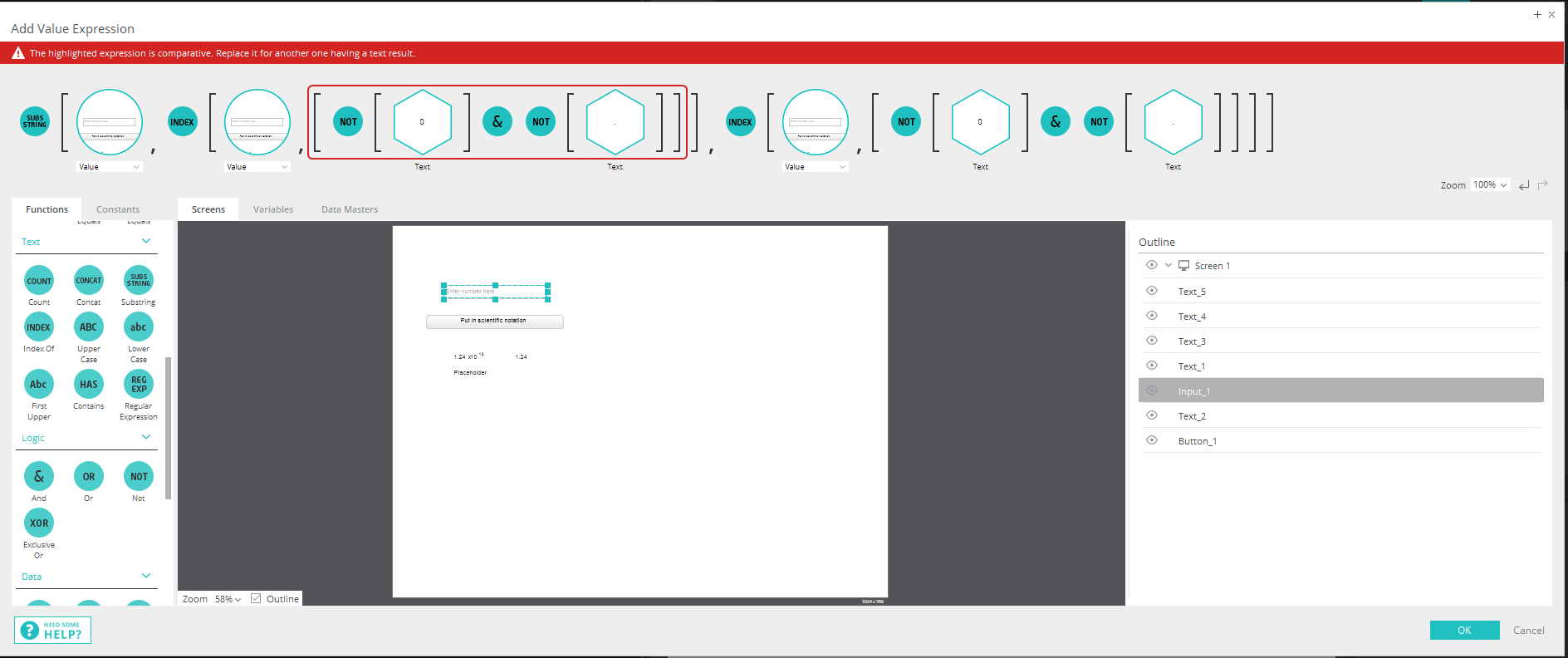I can't think of any other way to detect location of the first non-zero value in a value such as 0.0003.

Do anyone have a working prototype example that I can use?1

We found a way to do this using a variable and a looped 'on variable change' event1

Thank you very much for the quick reply.

The current function seems to be limited to the clean decimal numbers such as 0.000335. However, if the numbers are messier (such as 0.00033456) or larger (such as 0.335), the output would be incorrect or messy.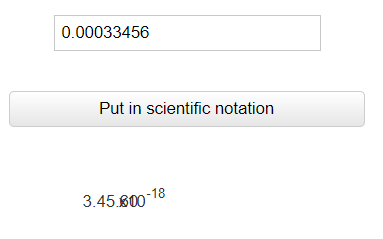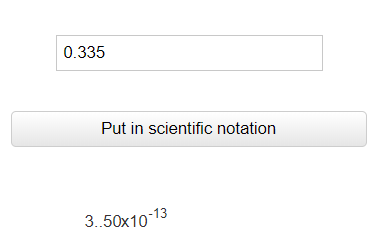Would it be possible to resolve these issues and limit output to 2 decimal points (like 3.35E-6)?1

Also, don't seem to work when on one digit is involved (like 0.003)?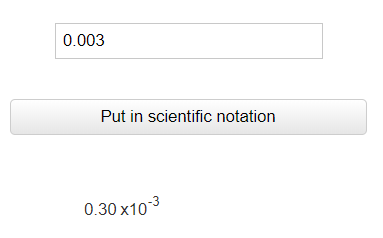1

I noticed that your prototype actually do convert value to scientific notations automatically if the value is too small. However, it only does conversion for values smaller than 17 decimals. Is there a way to limit that number of output value (ex. 1.25e-23) and increase that limit where the conversion occurs?